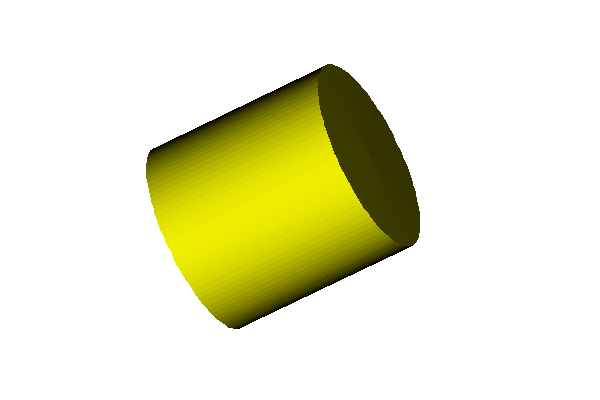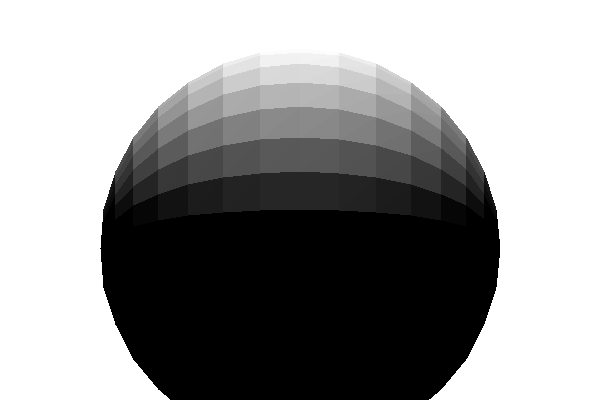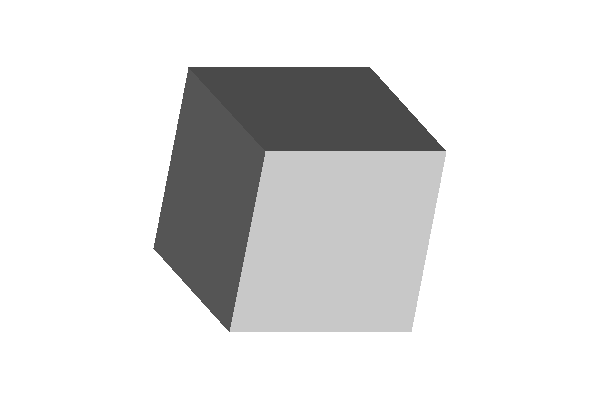25
28.04.2014

## Stage 3

Pracovna verzia. Konkretne zadanie stage sa este upresni. Vsetky informacie su zatial informacne

Hide invisible faces. Compute flat shading on the model.

Pracovna verzia. Konkretne zadanie stage sa este upresni. Vsetky informacie su zatial informacne

### 1. Implement back face culling

Hide invisible faces based on the angle between their normal vector and the vector towards camera. Remember, the camera is positioned in the axis and we use orthogonal projection.

Your application will have the following inputs:

• Light X, Light Y, Light Z. These will allow the user to input the position of the light (e.g. x = 3, y = 3, z = 5).
• Color R, Color G, Color B (or use a color selection dialog). This will define the object color (e.g. R = 255, G = 255, B = 0).

Compute diffuse light intensity in the center of every face. Use this light intensity to compute the color of the face:
Example:
object color = RGB(255, 255, 0)
intensity = 0.6
resulting color = RGB(153, 153, 0)

Use the color to draw a filled polygon of the face. You can use API function to draw the filled polygon.

### Examples:

Cylinder, RGB(255,255,0), Light (0,0,10):Sphere.obj , RGB(255,255,255), Light (0,10,0):Kocka.obj , 5x RotateX, 5x RotateY, RGB (255,255,255), Light (0,0,10)